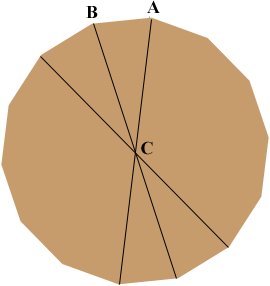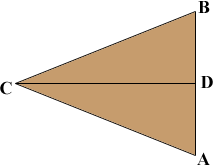SEARCH HOMEMath Central Quandaries & QueriesQuestion from Kenneth: I am 35 yr I am wanting to build a well house cover. I'm trying to figure out how long the pieces need to be and what angle they need to be for a 4 ft dia with 14 side well house. I would love an answer but would also like to know how to figure it in the future. Thanks KdKenneth,

Join each pair of opposite vertices to form 14 congruent triangles. One such triangle is ABC below.The angle BCA has measure 360/14 = 25.71 degrees.

I redrew triangle ABC and let D be the midpoint of AB so

the measure of angle BCD = 25.71/2 = 12.86 degrees.You said a 4 foot diameter so |CD| = 2 feet = 24 inches. Thus tan(12.86) = |DB|/|CD| and thus

|DB| = |CD| tan(12.86) = 24 × 0.2282 = 5.48 inches.

Thus

|AB| = 2 × 5.48 = 10.96 inches which is 10 15/16 inches.

I hope this helps,
HarleyMath Central is supported by the University of Regina and The Pacific Institute for the Mathematical Sciences.